## MOSFET Tester and Ohm Meter

1. Ohm Meter

Purpose: To use a DIP switch to switch between a mosfet tester and a ohm meter.

IO Devices used: 16x2 LCD panel, DIP Switch

## Ohm Meter¶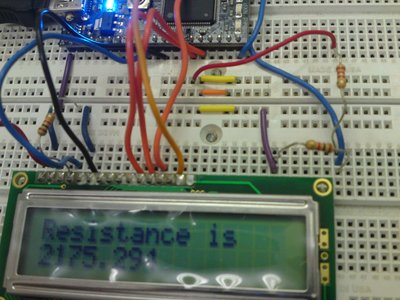Using the code below, the LCD screen will display the resistance and current flowing through a resistor. It was found to be accurate within about 60 Ohms.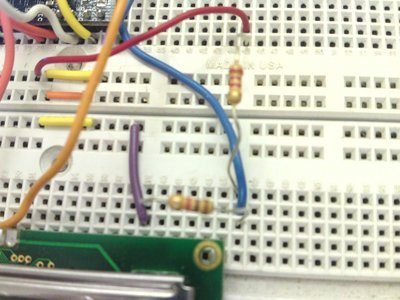Ohm Meter Technique: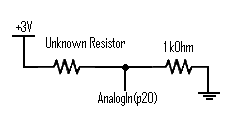• Run the 3.3V source (the red wire) through the unknown resistor (running vertical)
• Put the unknown resistor in series with another resistor (1 kOhm running horizontal) with a known value
• Connect the other end of this known resistor to ground.
• Run an analogIn(p20) from the node that is touching both the unknown resistor and the 1 kOhm resistor.

The Code for the Resistance Calculator:

#### main.cpp

```    //Declarations

//Resistance calculator
if (DIP4 && !DIP1) {
current = 3.3*V1/1000;
Voltage1 = 3.3*V1;
resistance = (3.3-Voltage1)/current;
lcd.printf("Current is \n%.6f\n", current);
wait(2);
lcd.printf("Resistance is \n%4.2f\n", resistance);
wait(2);
}
```

### MOSFET Tester¶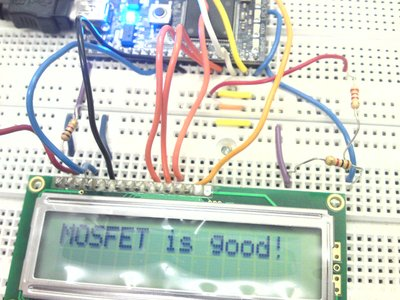Technique: Run the 3.3V source(with a pull-down resistor) into the Gate of the MOSFET. The AnalogOut pin is connected to the Source running 3.3V out of p18 (with a pull-down resistor). Connect an AnalogIn pin to the Drain.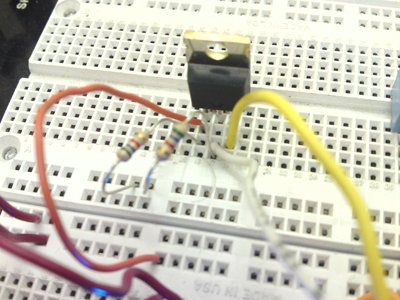#### main.cpp

```   //Declarations
AnalogOut TestVoltage(p18);
//MOSFET Tester
int main() {

t.start();
lcd.cls();
wait(1);

while(1) {
lcd.cls();
//MOSFET Tester
if (DIP1 && !DIP4) {
TestVoltage = 1.0f;
if (DSVoltage >= 1) {
lcd.printf("MOSFET is good!\n");
wait(2);
}
else {
lcd.printf("MOSFET no good!\n");
wait(2);
}
}
```